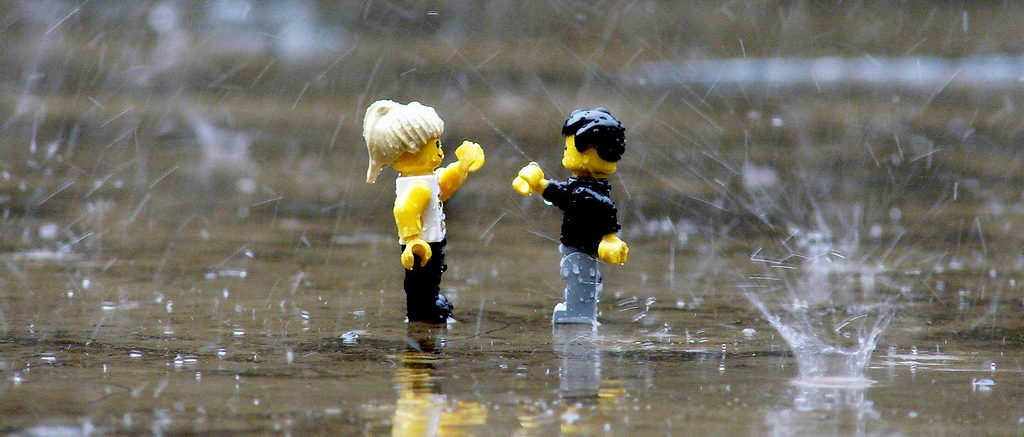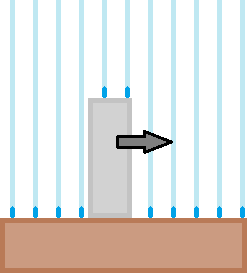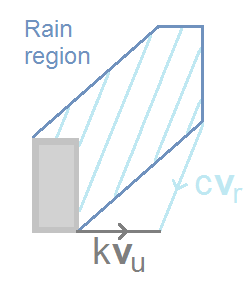# Is it better to run or walk in the rain?

When you’re caught in the rain without an umbrella… what is your best option?Image: Flickr user nur_h, cropped, CC BY-NC-SA 2.0

One of the many things affecting us living in the UK is the rain! Especially when we are caught out in it without an umbrella (or when we’re too lazy to dig it out of our bag). Intuitively it seems like a good idea to run, or at least walk faster so we spend less time in the rain, however this means that the rain hits our front at a faster rate. So what’s the best thing to do in order to minimise how wet you get? This problem has actually been discussed quite a bit in the past few decades, from mathematical journals to TV shows like Mythbusters (they actually did two episodes on this, the second being a correction!).Let’s start with the simplest model, imagine the rain coming straight down at a constant rate. In the figure to the left, you are represented as the grey rectangle (since the rain has made you sad). Suppose also that the raindrops fall uniformly and such that you cannot walk ‘into’ it, i.e. the rain is only hitting your head. In this case, regardless of the rate at which the rain is falling, your best option is to move as fast as you can to minimise your time spent in the rain.$k$ and $c$ are just positive constants here to show that the lines in the diagram are proportional to the actual vectors

Well, what if the rain is coming down towards you at an angle due to the wind? For this we’ll want to introduce some actual maths. Again, we will assume the rain is falling uniformly with a constant velocity $\textbf{v}_r$, which is a 2D vector with negative components. You are moving in the positive $x$-direction (i.e. to the right). An important concept in this problem is the rain region. This region contains the initial positions of all raindrops that will hit you at some future time.

Suppose you’re moving with speed $s$, so your velocity in 2D is $\textbf{v}_u=(s,0)$ where $s>0$ is scaled such that you spend the time $1/s$ in the rain. Let’s put a point $P$ on you and take a point $Q$ from the rain region, representing a rain drop that will hit you at time $t$. This rain drop will then hit you at position $Q+\textbf{v}_r t$. Your original point $P$ can be written as $P=Q+\textbf{v}_r t-\textbf{v}_u t$. Thus for every (exposed) point on you, $P$, the points $P+ (\textbf{v}_u -\textbf{v}_r)t$ are in the rain region for $t \in [0,1/s]$. The rain region is entirely made up of these lines, each with length $||\textbf{v}_u -\textbf{v}_r||/s$. It should be clear then if the rain is coming down at an angle, hitting your front, you should run as fast as you can (maximising $s$) to minimise the ‘length’ of the rain region (the ‘width’ of the rain region will be fixed, proportional to your height).

What if the rain is coming from behind you? Here things get a little more complicated. The components of the rain’s velocity are $\textbf{v}_r=(v_r^1,v_r^2)$ where $v_r^1>0$ since the rain is falling in the forwards direction, $v_r^2$ is always negative. A few distinct things can happen here (with our assumption of the rain falling uniformly, at the same velocity). Recall that you move with speed $s$.

• If $s>v_r^1$ you will overtake the rain falling from behind you and only your top and front gets wet.
• If $s=v_r^1$ you are moving with the rain and only the top of you gets wet.
• If $s<v_r^1$ the rain hits your back and top, but you do not walk into any rain so your front remains dry.

Let $A_{fb}$ be the area of your front or back exposed to the rain, and $A_{t}$ is the area of the top of your head. In our 2D case, these would just be the height and width of your ‘rectangle’, respectively. The amount of rain hitting these parts are then proportional to $R_{fb}=|v_r^1-s|A_{fb}$ and $R_{t}=|v_r^2|A_{t}$ (again, respectively). Recalling that the time spent in the rain is $1/s$, the total wetness function, $R$, is proportional to:
\begin{align} R_1(s)=\frac{1}{s}[ (v_r^1 – s) A_{fb} + |v_r^2| A_{t} ]; \quad \text{if } s\leq v_r^1 \nonumber \\
R_2(s)=\frac{1}{s}[ (s-v_r^1) A_{fb} + |v_r^2| A_{t}]; \quad \text{if }  s\geq v_r^1
\end{align}
where the multiplier for the proportion is just the density of the rain. Note that this function can be applied to the previous case too where the rain is falling backwards, into you ($v_r^1<0$). For that case, we always have $R=R_2$ and the way this is minimised is to increase $s$ as much as possible.

For the case of the rain falling forwards ($v_r^1>0$). Let $C=-v_r^1 A_{fb} + |v_r^2| A_{t}$ and notice that $R$ is continuous, $R_1$ is a decreasing function of $s$, and that the behaviour of $R_2$ depends on the sign of $C$.

• If $C>0$, $R_2$ is also a decreasing function of $s$ and $R$ will be minimised when $s$ is increased to its maximum.
• If $C=0$, then $R_2$ is a constant and we can minimise $R$ by taking any $s\geq v_r^1$.
• If $C<0$, $R_2$ is an increasing function of $s$, so we can only minimise $R$ by taking $s=v_r^1$, i.e. you run at exactly the horizontal speed of the rain.

If the rain is only slightly falling forwards, meaning $v_r^1$ is small enough such that $C>0$, then $R$ is a decreasing function in $s$ and your best option will still be running as fast as you can! However, if the rain is falling forwards by a decent amount (such that $C<0$), then you’re better off running at exactly the horizontal speed of the rain where $R$ has its minimum value. This also means the rain will only be hitting your head (theoretically)!

There are many more complicated models for this, taking into account things like different shapes (other than rectangles) or gusts of wind which affect the final conclusions.  Instead, we’ll just end on a limerick by Matthew Wright (unfortunately, not the previous member of Chalkdust)!

When caught in the rain without mac,
walk as fast as the wind at your back.
But when the wind’s in your face,
the optimal pace
is fast as your legs will make track.

In many of these related articles, you’ll find this limerick as a longer poem, adjusted to include the new results! For example, here is one by Dan Kalman and Bruce Torrence (or as they called themselves, Dank Hailman and Bruce Torrents):

When you find yourself caught in the rain,
while walking exposed on a plane,
for greatest protection
move in the direction
revealed by a fair weather vane.
Moving swift as the wind we’ll concede,
for a box shape is just the right speed.
But a soul who’s more rounded
will end up less drownded
if the wind’s pace he aims to exceed.Atheeta is a PhD student at UCL in the area of mathematical ecology. He also has interests in evolutionary game theory and social dynamics.